Published: 30 September 2013

# Ensemble learning-based intelligent fault diagnosis method using feature partitioning

Yongsheng Zhu1
Xiaoran Zhu2
Jing Wang3
1, 2, 3School of Mechanical Engineering, Xi’an Jiaotong University, Xi’an 710049, China
Corresponding Author:
Jing Wang
Views 48

#### Abstract

Diversity and fusion strategy are the key factors which affect the performance of the ensemble learning systems. In this paper, to tackle the neighborhood factor selecting difficulty of the traditional neighborhood rough set method, the wrapper feature selection algorithm based on kernel neighborhood rough set is introduced to find a set of feature subsets with high diversity, and then a base classifier selection method is proposed for constructing the ensemble learning systems. To increase the diversity, the heterogeneous ensemble learning algorithm based on the proposed base classifier selection method is designed and compared with the similar homogeneous ensemble learning algorithm. To study the effect of the fusion strategy on the final performance of the ensemble learning system, majority voting and D-S theory for fusing the outputs of base classifiers of ensemble learning system to get final decision are compared experimentally. The results on UCI data and the fault signals of rotor-bearing system show that the heterogeneous ensemble learning system with D-S fusion strategy can get the best classifying performance, and the ensemble learning system is superior to single classification system in most cases.

## 1. Introduction

The essence of mechanical equipment fault diagnosis is the pattern recognition, therefore various intelligent classification methods have been developed and adopted to tackle this problem [1, 2]. Recently, the ensemble learning system, which is proved to have better performance than the single classifier, is attracted increasing attention and is applied successfully to the field of fault diagnosis [3, 4]. Although various classifiers for ensemble models have been proposed and studied, pursue on ensemble learning algorithm of higher performance is still a keep-on-going research topic.

The performance of the ensemble learning system is supposed to be better than the single classifier , since the ensemble learning system combines the outputs of the several independent base classifiers with a fusion strategy to make the final decision [6, 7] and therefore the mis-decision risk is reduced. Empirically, the ensemble learning system tends to yield better results when there is a significant diversity among the base classifiers. Two methods: data partitioning method and feature partitioning method are normally used to construct the single base classifiers for ensemble system. The data partitioning method, which is to train base classifiers with different sample subsets, mainly includes the bagging  and boosting [9, 10]. However, the performance of data partitioning method decreases significantly when the dataset contains small samples. Actually, this is the case in mechanical fault diagnosis field, where the fault samples are always insufficient. In contrast, various kinds of features can be obtained easily from each sample since the development of the signal processing technology, and therefore enough number of features can be prepared even though only limited numbers of samples are available. For this reason, the feature partitioning method is expected to achieve better results and is more preferable for fault diagnosis applications than data partitioning method.

Feature partitioning method divides the feature set into a set of feature subsets, and then combines the results of base classifiers that are trained with the feature subsets. For example, in 1998, the random subspace method was introduced by Ho . In 2003, Robert Bryll et al. proposed a feature bagging based on the random subsets of features . In 2005, Oliveira et al. proposed an ensemble feature selection approach based on the hierarchical multi-objective genetic algorithm . Intuitively, the feature partitioning method tries to produce diversity via using different feature subsets, so the key factor affecting the performance of feature partitioning method is how to generate a set of feature subsets which do not lose the distinguishing information  while keeping the good diversity properties. Normally, the subsets presented to multiple classifiers may be produced by employing the feature selection method . A well-designed feature selection algorithm would significantly improve the performance of feature partitioning ensemble system. The rough set (RS, rough set), which was proposed by Pawlak , has attracted much attention from machine learning and data mining fields and recently introduced in ensemble learning system [17, 18]. In the view of RS, different reducts can be generated from the initial feature set by adjusting the controlling factor of RS. The reducts are in fact the feature subsets which keep the approximation ability of the initial total features but contain less number of features. So, the base classifiers trained with the obtained reducts, are expected to improve or maintain the similar classification performance as the classifier trained with the initial features. In addition, the difference among reducts can provide diversity among the obtained base classifiers. Therefore, feature partitioning ensemble system based on rough set is supposed to obtain better generalization ability.

Several ensemble learning systems based on RS have been proposed. For example, Suraj et al. proposed an approach of multiple classifier system using RS to construct classifier ensemble . In the method, the different combinations of selected reducts but not the recudts themselves were used to train base classifiers, the results show that in most cases, the ensemble systems get better performance than the single classifier system, however, the selection algorithm of reducts is said by author to be complex. In reference , Qinghua Hu et al. proposed a FS-PP-EROS algorithm for selective ensemble of rough subspaces. In Hu’s method, each obtained reduct was used to train a classifier and then the classifiers were sorted by the accuracy. To construct the ensemble system, the classifier was added into the ensemble system sequentially and the classification performance during the addition was recorded. Finally, the post-pruning is conducted by eliminating the base classifiers which are added after the peak accuracy. Since the performance curve fluctuates with the adding of the base classifiers, the proposed method only eliminated the base classifiers after the peak while kept the base classifiers which cause the performance fluctuation before the peak, and the final performance and efficiency of the obtained ensemble system are possibly affected strongly by these unwanted classifiers.

On the other hand, the traditional Pawlak’s RS method was originally proposed to deal with categorical data, and the numerical data such as the features of vibration signals of the mechanical equipment must be reduced by using the Pawlak’s RS method after the discretization. However, some important information may be missed from numerical features after discretization . In order to directly deal with the numerical data, the neighborhood rough set was proposed [22, 23, 24]. In practice, it was discovered that the neighborhood value has serious influence on the reducts. In our previous work, the feature selection algorithm based on kernel neighborhood RS method was proposed to solve this problem . In the method, the kernel method and neighbor rough set are combined to design the wrapper feature selection algorithm, and the neighborhood factor was calculated by mapping the data to a high-dimension feature space via the Gaussion kernel function and calculating the hypersphere radius as the neighborhood value. The experimental results shown that the proposed method can obtain reduct of better performance more easily than the traditional RS method. Also, by changing the hypersphere radius, a set of reducts of numerical features may be generated by the kernel neighborhood RS method, and the ensemble learning system based on the kernel neighborhood RS method could be constructed.

In this paper, a new selection method of the base classifiers is designed and applied to both heterogeneous ensemble and heterogeneous ensemble learning systems. At the same time, two fusion strategies of base classifiers (majority voting and D-S theory) are tested. First, the kernel neighborhood RS method is adopted to obtain feature subsets. Then the homogeneous ensemble algorithm and heterogeneous ensemble algorithm, which are proved to have different classification performance, are designed based on the optimal feature subset and the obtained a set of sub-optimal feature subsets. In both algorithms, the subsets are added into the ensemble learning system one by one, and the performance of the ensemble learning system is evaluated for each addition. The base classifier which contributes to the performance increasing is kept while the one which causes the performance decreasing is ignored. Third, to improve the final classifying accuracy furthermore, the majority voting and D-S theory fusion strategies of ensemble system which are applicable to different types of classifier’s outputs of label information and probability information are compared.

The rest of the paper is structured as follows. Basic information about feature selection based on kernel neighborhood rough set is given in Section 2. Section 3 presents the ensemble algorithm. The analysis results are shown in Section 4 and Section 5. The conclusion comes in Section 6.

## 2. Feature selection based on kernel neighborhood rough set

The basic idea of the feature selection based on kernel neighborhood rough set is mapping datasets into the high-dimensional space using the kernel function. The smallest hypersphere, which contains all datasets, is obtained. The hypersphere radius $R$ may be considered the maximum neighborhood value for $\delta$. Moreover, $R$ is considered the upper bound for $\delta$. The gaussian kernel function is adopted:

1
$K\left(x,y\right)=\mathrm{e}\mathrm{x}\mathrm{p}\left(-\frac{{‖x-y‖}^{2}}{2{\sigma }^{2}}\right).$

The kernel parameter value $\sigma$ affects the hypersphere. Thus, the wrapper feature selection algorithm is designed with $\sigma =$ 3 to 4.5 with step 0.05 to determine a set of feature subsets. The feature selection algorithm is shown as Fig. 1. For more detailed information on this algorithm, please review .

Fig. 1The flow chart of the feature selection algorithm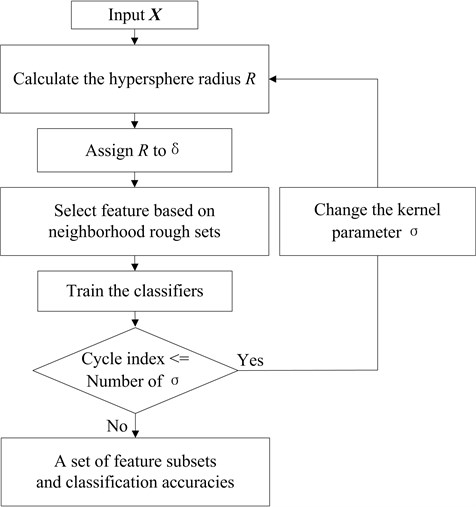## 3. Ensemble learning algorithms design

### 3.1. Homogeneous ensemble algorithm

According to the feature selection algorithm introduced in section 2, when the kernel parameter changed, the resultant feature subset might be changed. By using different kernel parameters, a set of feature subsets can be obtained. The feature subsets have two important characteristics. (1) Each of the feature subsets can improve or maintain the classification ability since some redundant or even noised features are ignored during feature selection process. (2) The feature subsets have diversity with each other because each of the feature subsets includes different features. Therefore, the homogeneous ensemble algorithm could be designed as follows. First of all, a series of base classifiers are trained with a set of feature subsets and the corresponding classification accuracies are recorded, then the feature subsets are sorted with descending order according to the classification accuracies. In the first ensemble step, the feature subset with the highest accuracy is selected, and then the next feature subset from the rest in each stage is selected and is added into the homogeneous ensemble algorithm. If the accuracy of the homogeneous ensemble algorithm is improved, the new added feature subset is retained; otherwise, the feature subset is deleted. Finally, a set of optimal feature subsets and the optimal classification accuracy of the homogeneous ensemble algorithm can be obtained. The homogeneous ensemble algorithm is shown as Fig. 2.

Fig. 2The flow chart of the homogeneous ensemble algorithm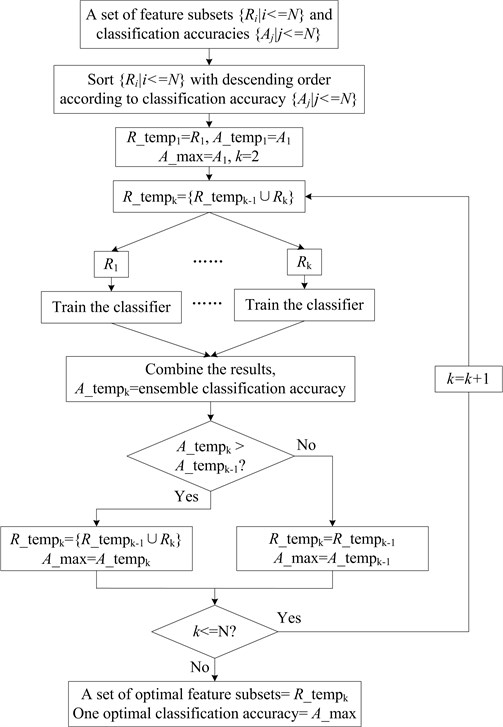### 3.2. Heterogeneous ensemble algorithm

The objective of the heterogeneous ensemble algorithm is to reduce the uncertainty and inaccuracy in classification problem by using the complementary of different base classifiers. In this paper, the basic idea of the heterogeneous ensemble algorithm is very similar to the homogeneous ensemble algorithm, except for that the heterogeneous ensemble system needs two or more base classifiers to integrate. The feature partitioning method refers to making use of the instability of the base classifier and the neural network is subject to the influence of the initialization. Therefore, the BP network and the RBF network are chosen as the base classifier. The heterogeneous ensemble algorithm is shown as Fig. 3.

Fig. 3The flow chart of the heterogeneous ensemble algorithm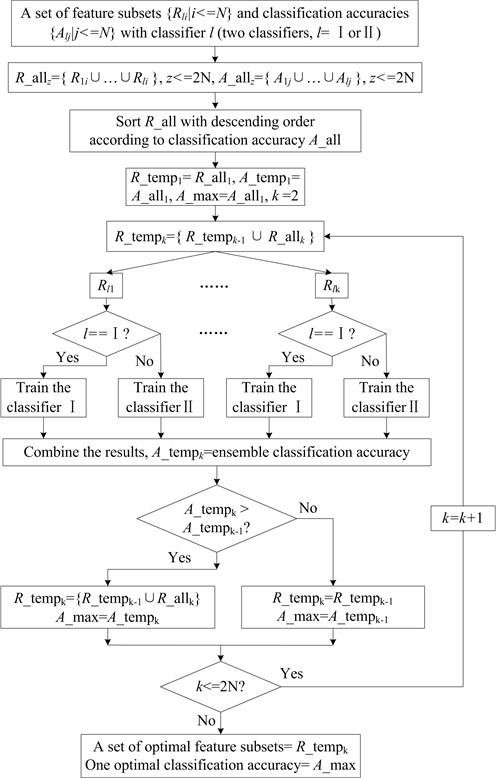### 3.3. Base classifier and fusion strategy

How to select fusion strategy according to the output type of the base classifier is an important study on ensemble learning system. Generally speaking, the output type of the base classifier is classified into two main categories: label information and probability information . In this paper, two types of base classifiers, the BP network and the RBF network, are used. The label information or the probability information can be exported for neural network. With considering the output type of the base classifier, selecting the suitable fusion strategy will be a good solution. In this paper, the majority voting is chosen as the fusion strategy for label information and the D-S theory is chosen as the fusion strategy for probability information.

## 4. Algorithm verification with UCI datasets

The datasets (Diab, Iono, Sonar, Wine, Wpbc) from University of California at Irvine (UCI)  are firstly chosen to test the proposed ensemble learning system. The UCI datasets information is shown in Table 1 and the features are normalized into the interval $\left[0;1\right]$. The feature selection algorithm based on the kernel neighborhood rough sets, which is described in section 2, is applied to the UCI datasets to select features for each dataset. The number of selected features and the corresponding classification accuracy of two kinds of base classifiers are shown in Fig. 4.

Fig. 4Variation of classification accuracies and numbers of selected features with hypersphere radius R on a) Diab, b) Iono, c) Sonar, d) Wine and e) Wpbc dataset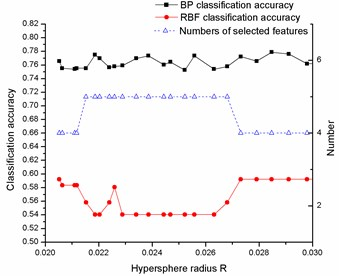a)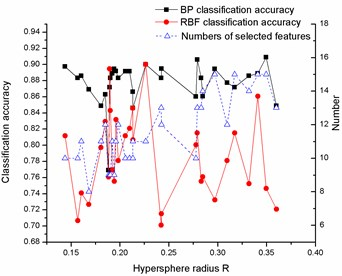b)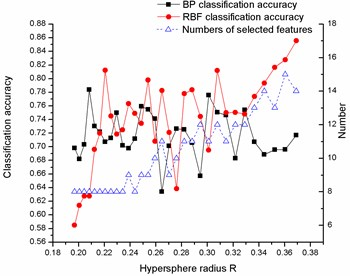c)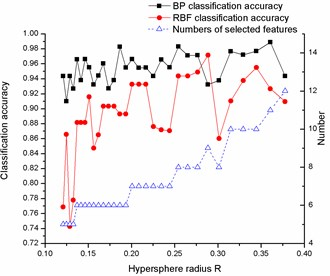d)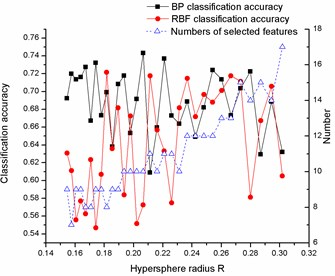e)

Table 1UCI dataset descriptions

 Datasets Samples Features Classes Diab 768 8 2 Iono 351 34 2 Sonar 208 60 2 Wine 178 13 3 Wpbc 198 33 2

Fig. 4 shows that for all datasets, the classification accuracies and the numbers of the selected features vary with the different hypersphere radius. Meanwhile, the numbers of the feature subsets are much less than before, it means that the redundant features can be efficiently deleted by using the feature selection algorithm. Another interesting phenomenon is that even for the same number of feature subsets, the obtained classification accuracies are different apparently, this result further illustrated that the feature subset exerts a serious influence on classification accuracies, and the proposed feature selection algorithms can produce feature subsets of high diversity.

The diversity among the base classifiers also plays an important role in constructing an ensemble learning system. By observing the results shown in Fig. 4, one can easily find that for each dataset, the diversity exists among the same kind of base classifiers which are trained with the different feature subsets, so the homogeneous ensemble algorithm could be developed based on these observation and a better classification performance could be expected. On the other hand, the big differences of the classification accuracy between two types of base classifiers and the fluctuation with the subsets suggests the possibility of improving classification performance by applying the heterogeneous ensemble algorithm.

The ensemble algorithms are conducted on UCI datasets and the results are summarized in Fig. 5. For each dataset, to show the effects of feature selection, the BP network and the RBF network are firstly trained with the original dataset and the selected subsets separately; and then the homogeneous ensemble algorithm is applied to each kind of networks and the heterogeneous ensemble algorithm is applied to combine the results of BP and RBF networks. In each ensemble experiment, both the majority voting and the D-S fusion strategies are tested.

Fig. 5 shows that for the single base classifier, BP networks get better classification accuracy than RBF networks, and the selection process of features improves the classification ability of the base classifier apparently.

Fig. 5Comparison of the classification accuracies for the UCI datasets a) Diab, b) Iono, c) Sonar, d) Wine and e) Wpbc dataset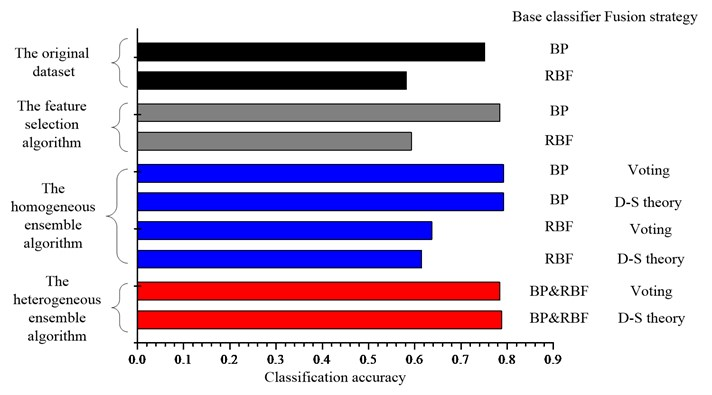a)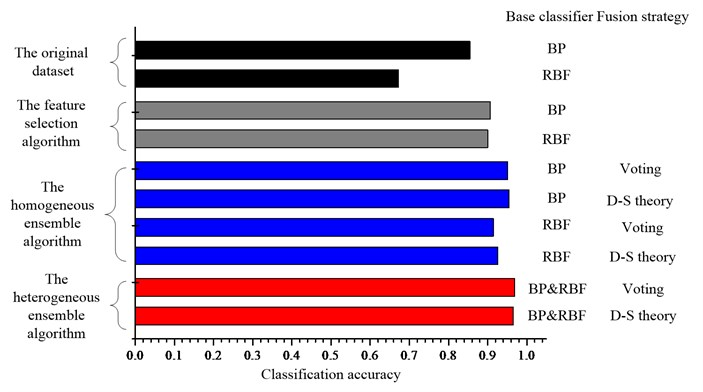b)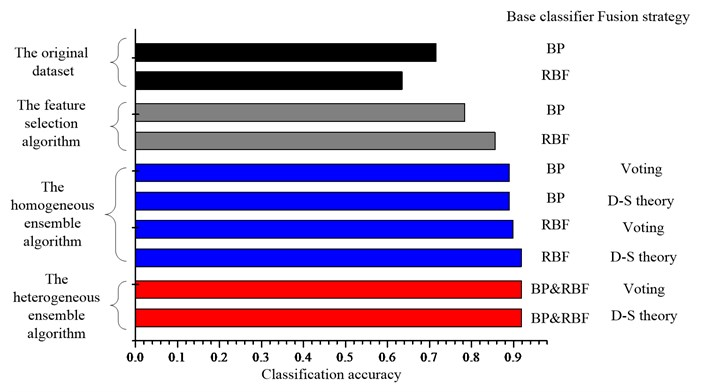c)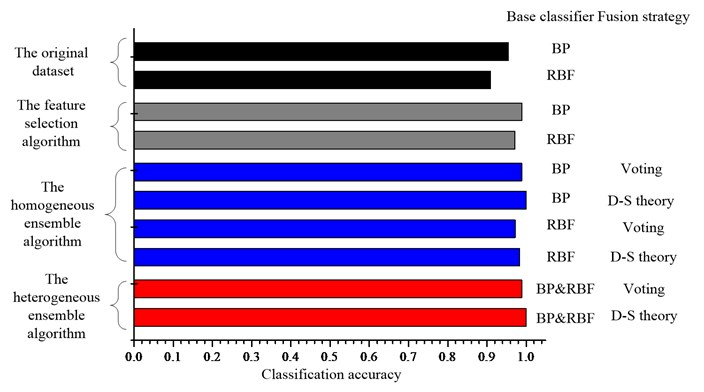d)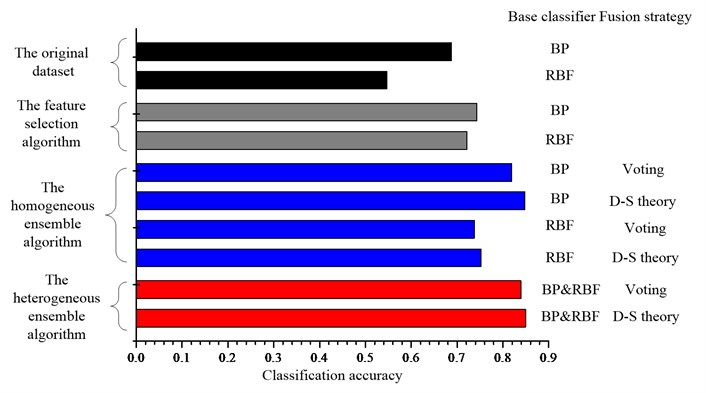e)

The ensemble learning method, no matter the homogeneous ensemble algorithm or the heterogeneous ensemble algorithm, can improve the classification performance further compared with the single classifier for most datasets. And the performance of base classifiers has strong effect on the final accuracy of ensemble system. For example, if the base BP network has superior performance, the ensemble of BP networks will give a better classification performance than RBF networks and vice versa. By comparing the performance of two types ensemble learning algorithms, one can conclude that as expected, the heterogeneous ensemble algorithm can gain a better classification performance than the homogeneous ensemble algorithm, since it use not only the diversity induced by different feature subsets, but also the diversity existing between the different classifiers.

As for the influence of the fusion strategy on the final classification accuracy, as shown in the Fig. 5, for the homogeneous ensemble, the results indicate that the D-S theory can gain a better classification performance than the majority voting except for the Diab dataset. For the heterogeneous ensemble, the results indicate that the D-S theory can gain a better classification performance except for the Iono dataset. Since D-S theory is applied to the probability information while the majority voting method is applied to the label information, the results suggest that combining the probability information should be better than combining the label information.

## 5. Experimental result

The test rig layout is shown in Fig. 6. The test rig is driven by a DC motor. A flexible coupling was used between the shaft and the motor to isolate the vibration transmitted from the motor. Some eddy current displacement sensors were mounted on different positions and acquired the vibrations information in horizontal and vertical directions, respectively. In the experiments, the vibration data was collected in four different states of the rotor system, including normal, rubbing, unbalance and misalignment (by adjusting the eccentric bearing) state. The rotational speed is set as 300 r/min.

Because the statistical features of vibration signal contain an amount of information and is very efficient in calculation, the intelligent fault diagnosis based on statistical features has received increasing attention [3, 30]. In this paper, six kinds of statistical features (as shown in Table 2) are extracted from both time domain and frequency domain. To extract features in time domain, raw vibration signals are filtered into low frequency band ($\le 0.49{f}_{0}$, where the ${f}_{0}$ is rotation frequency), medium frequency band $\left(0.5{f}_{0}~1.5{f}_{0}\right)$ and high frequency band $\left(\ge 1.51{f}_{0}\right)$ by using filters, and are decomposed into 3 levels with wavelet packet transform (WPT, and the wavelet basis function is db3), and then six statistical features are extracted for each band, each decomposed signal and the raw signal respectively. To extract the features in frequency domain, the power spectrums are calculated for above-mentioned three filtered signals and the original signals, and then six statistical features are extracted from every power spectrum respectively. Finally total 96 features can be obtained. The information about the fault dataset (the dataset name is DALL) is shown in Table 3.

Fig. 6The test rig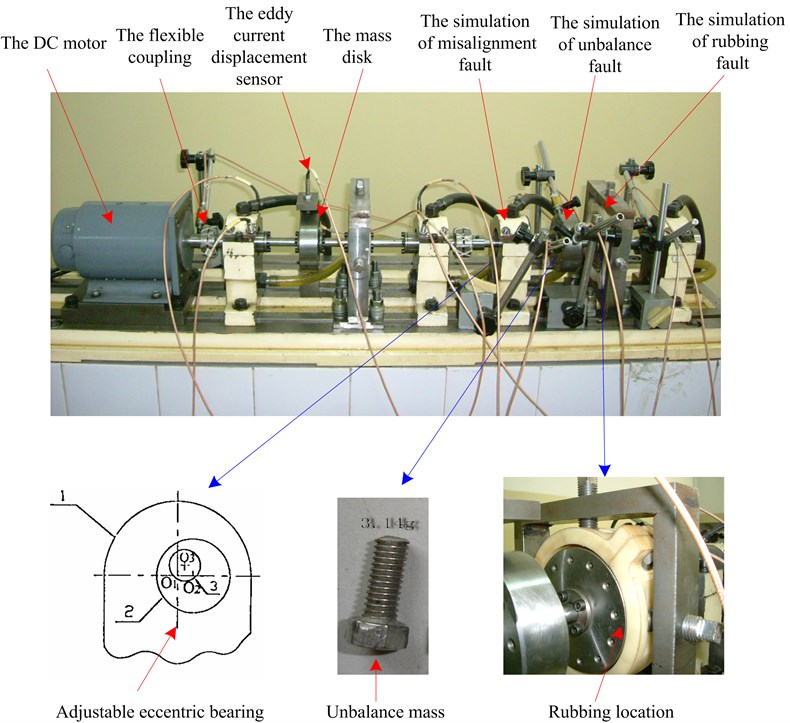Table 2Statistical features

 Feature Equation Feature Equation Skewness ${x}_{ske}=\frac{1}{n}\sum _{t=1}^{n}{\left(x\left(t\right)-\stackrel{-}{x}\right)}^{3}$ Kurtosis ${x}_{kur}=\frac{1}{n}\sum _{t=1}^{n}{\left(x\left(t\right)-\frac{1}{n}\sum _{t=1}^{n}x\left(t\right)\right)}^{4}$ $I$ factor $I=E\left\{\mathit{max}\left(\left|x\left(t\right)\right|\right)\right\}/\frac{1}{n}\sum _{t=1}^{n}x\left(t\right)$ $S$ factor $S={\left(\frac{1}{n}\sum _{t=1}^{n}{x}^{2}\right)}^{1/2}/\frac{1}{n}\sum _{t=1}^{n}x\left(t\right)$ $C$ factor $C=E\left\{\mathit{max}\left(|x\left(t\right)|\right)\right\}/{\left(\frac{1}{n}\sum _{t=1}^{n}{x}^{2}\right)}^{1/2}$ $L$ factor $L=E\left\{\mathit{max}\left(\left|x\left(t\right)\right|\right)\right\}/{\left(\frac{1}{n}\sum _{t=1}^{n}{\left|x\right|}^{1/2}\right)}^{2}$

Fig. 7The flow chart of the fault dataset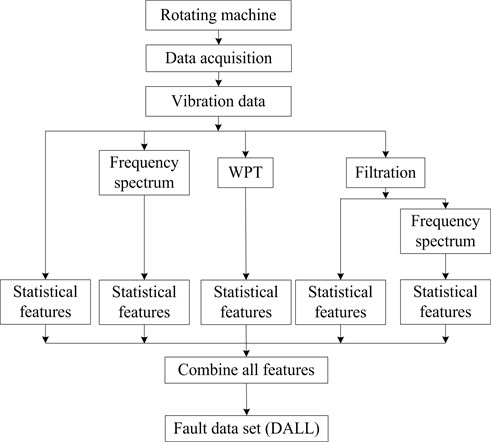Table 3The fault dataset DALL description

 Dataset Samples Features Fault type Classification label DALL 30 96 Normal 1 30 96 Rubbing 2 30 96 Unbalance 3 30 96 Misalignment 4

The feature selection algorithm is applied to the DALL datasets. The result is shown in Fig. 8. The trend of classification accuracy changing is similar to UCI datasets. It is found that unrelated features are substantively deleted.

Fig. 8Variation of classification accuracies and numbers of selected features with hypersphere radius R on the DALL dataset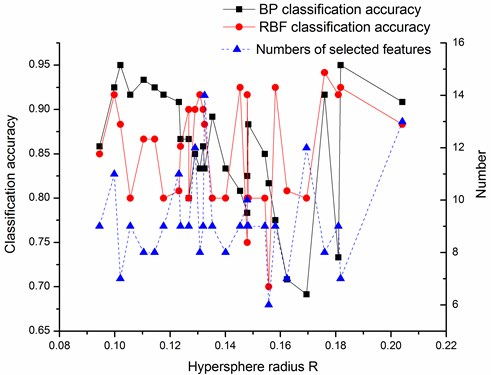When FFT, WPT and filters are used to process the vibration signal, the obtained 96 features include not only the time-domain information, but also the frequency-domain information. Obviously, it is possible that some unrelated features are also acquired during the processing. Namely, not all features make contributions to the faulty identification; some of them are insensitive to distinguish the faults. Therefore, the classifiers trained with all features would be confused and the classification accuracy is possibly low. However, when the sensitive features are selected by using the feature selection algorithm, the classification accuracy is improved greatly. To further improve the classification accuracy, the ensemble learning systems that are proposed in this paper are conducted on the DALL dataset, the results are shown in Fig. 9.

Fig. 9Comparison of the classification accuracies for the DALL dataset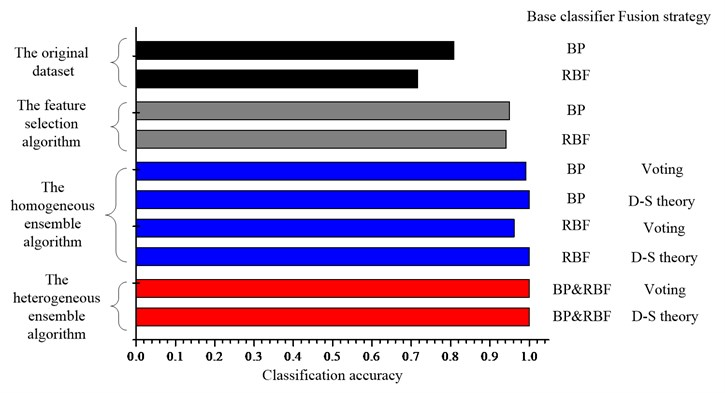The classification accuracy is improved from 0.7624 (average) to 1 (average) by adopting the ensemble learning algorithms, it means that the proposed ensemble intelligent fault diagnosis approach can diagnose different faults of the rotor-bearing system accurately. From the results, we get the similar conclusion as the above: ensemble learning can improve the classification accuracy; the D-S theory seems to be the powerful fusion tool.

## 6. Conclusions

In order to improve the classification accuracy, the ensemble learning systems based on the feature partitioning are proposed. First, the homogeneous ensemble algorithm and the heterogeneous ensemble algorithm that both are based on the feature selection of the kernel neighborhood rough set are designed. Second, the majority voting is chosen as the fusion strategy for label information and the D-S theory is chosen as the fusion strategy for probability information. Third, the UCI datasets and the fault dataset DALL are used to test the classification performance of the ensemble learning systems. The results show that: (1) the ensemble learning systems can improve the classification performance and the heterogeneous ensemble algorithm can gain a better classification performance than the homogeneous ensemble algorithm. (2) Because probability information can provide more complementary information, the fusion strategy of the D-S theory can gain a better classification performance than the majority voting. (3) The application results of the rotating machinery demonstrate the effectiveness of the presented fault approach.

Automatic identifying the running condition of machine is supposed to be the developing trend of fault diagnosis technology, and it is also found by many researchers to be difficult to find one feature superior to the others in many cases. In this paper, a feature selection method is introduced and the fault identifying accuracy is guaranteed by designing homogeneous and heterogeneous ensemble learning systems. The improvement on the classification accuracy is attributed to the diversity among subsets of features and base classifiers, as well as the good fusion strategy.